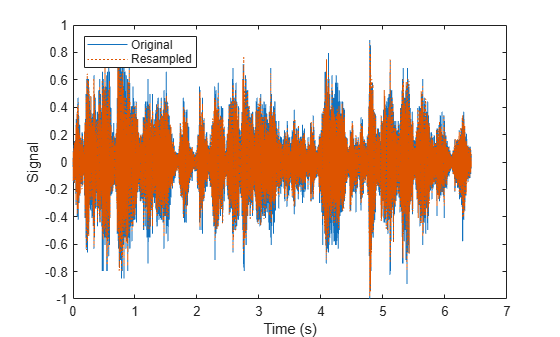# partition

Partition signal datastore and return partitioned portion

## Syntax

``subsds = partition(sds,numPartitions,index)``
``subsds = partition(sds,'Observations',index)``
``subsds = partition(sds,'Observations',obsname)``

## Description

example

````subsds = partition(sds,numPartitions,index)` partitions the signal datastore into the number of parts specified by `numPartitions` and returns the partition corresponding to `index`.```

example

````subsds = partition(sds,'Observations',index)` partitions the signal datastore and returns the partition corresponding to the `index` in the `Observations` property. If `sds` contains file data, the function partitions the signal datastore by files.If `sds` contains in-memory data, the function partitions the signal datastore by members. ```
````subsds = partition(sds,'Observations',obsname)` partitions the signal datastore and returns the partition corresponding to the observation name `obsname`. If `sds` contains file data, the function partitions the datastore by files.If `sds` contains in-memory data, the function partitions the datastore by members. ```

## Examples

collapse all

Specify the file path to the example signals included with MATLAB®. Create a signal datastore that points to the specified folder.

```folder = fullfile(matlabroot,'toolbox','matlab','audiovideo'); sds = signalDatastore(folder,'SampleRateVariableName','Fs');```

Get the default number of partitions for the signal datastore.

`n = numpartitions(sds)`
```n = 7 ```

Partition the datastore into the default number of partitions and return the datastore corresponding to the fourth partition.

`subsds = partition(sds,n,4);`

Use the `extractAfter` function to display the name of the file contained in the datastore corresponding to the fourth partition.

`fName = extractAfter(subsds.Files,'audiovideo\')`
```fName = 1x1 cell array {0x0 char} ```

Read the data and information about the signal in the datastore corresponding to the fourth partition. Extract the sample rate from `info` and resample the signal to half the original sample rate. Plot the original and resampled signals.

```while hasdata(subsds) [data,info] = read(subsds); fs = info.SampleRate; f_res = 0.5*fs; ts = (0:length(data)-1)/fs; data_res = resample(data,1,2); t_res = (0:length(data_res)-1)/f_res; plot(ts,data,t_res,data_res,':') xlabel('Time (s)') ylabel('Signal') legend('Original','Resampled','Location','NorthWest') end```Specify the path to a directory containing example signals included with MATLAB®.

`folder = fullfile(matlabroot,'toolbox','matlab','audiovideo');`

Create a signal datastore that points to the specified folder.

`sds = signalDatastore(folder);`

Return an estimate for a reasonable number of partitions for parallel processing, given the current parallel pool.

`pool = gcp;`
```Starting parallel pool (parpool) using the 'local' profile ... Connected to the parallel pool (number of workers: 6). ```
`n = numpartitions(sds,pool)`
```n = 7 ```

Partition the signal datastore and read the signal data in each part.

```parfor ii = 1:n subds = partition(sds,n,ii); while hasdata(subds) data = read(subds); end end```

## Input Arguments

collapse all

Signal datastore, specified as a `signalDatastore` object.

Number of partitions, specified as a positive integer. Use the `numpartitions` (Audio Toolbox) function to estimate a reasonable value for `numPartitions`.

Data Types: `single` | `double`

Index of sub-datastore, specified as a positive integer in the range `[1,numPartitions]`.

Data Types: `single` | `double`

Observation name, specified as a string scalar or a character vector.

The value of `obsname` is:

• A file name in the case of file data.

• A member name in the case of in-memory data.

Data Types: `char` | `string`

## Output Arguments

collapse all

Output signal datastore, returned as a `signalDatastore` object.